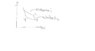# Need help in thermodynamics: Calculations for a thermal cycle

michealyap

## Homework Statement

how to know the maximum and minimum temperature for cycle in.
Carnot thermal efficiency 60%
heat transfer during isothermal expansion 40kj
pressure is 5.6bar and volume is 0.3m^3

## Homework Equations

One kilogram of air as an ideal gas executes a Carnot power cycle having a thermal efficiency of 60%. The heat transfer to the air during the isothermal expansion is 40 kJ. At the end of the isothermal expansion, the pressure is 5.6 bar and the volume is 0.3 m³.

(a) Sketch the cycle on p-v coordinates.
(b) Determine the maximum and minimum temperatures for the cycle, in K.
(c) Determine the pressure and volume at the beginning of the isothermal expansion in bar and m³, respectively.
(d) Determine the work and heat transfer for each of the four processes, in kJ

## The Attempt at a Solution

i know how to sketch the pv coordinates diagram however i am struggling to find the maximum and minimum T.

#### Attachments

•Untitled.jpg
8.7 KB · Views: 628
Last edited by a moderator:

Homework Helper
Gold Member
You have marked four states (1,2,3,4) in your p-v diagram, which state do you believe has the higher temperature and which state has the lower temperature?

Might help you the fact that because process 1->2 is isothermal that means that state 1 and 2 have the same temperature ##T##. Similar for the process 3->4, states 3 and 4 have the same temperature ##T'##.

michealyap
state 1 has the highest temperature. yeah i know t1=t2 and t3=t4. i understand that that i am stuck. we know at point 2 pressure is 5.6bar and volume is 0.3m^3. isothermal means same temperature same pressure as well? W=-pdeltv.

Homework Helper
Gold Member
state 1 has the highest temperature. yeah i know t1=t2 and t3=t4. i understand that that i am stuck. we know at point 2 pressure is 5.6bar and volume is 0.3m^3. isothermal means same temperature same pressure as well? W=-pdeltv.
Ok correct state 1 has the highest temperature. From the data given you can calculate (using the ideal gas law) the temperature in state 2, which is the same as the temperature of state 1.

For the temperature of state 3(or 4) use the fact that the thermal efficiency is ##0.6=1-\frac{T_1}{T_4}##

And no, during an isothermal expansion neither the pressure or the volume remains the same, its only the temperature that remains the same.

michealyap
pv=mrt. dry air = 28.97 g/m so i take 1000g/28.97g = 34.518 mol .. 560000 [pa] * 0.3 [m^3] = 34.518mol * 8.314 * T. solve the equation i got .. T1 = 312C then using carnot cycle efficiecy 0.6=1−T1/T4. i got 124.8C.

For question C) how to do? i know p1v1=p2v2 . we can use w=pdeltaV to get the initial v1. then use p1v1=p2v2 to solve.am i right in the approach.

Homework Helper
Gold Member
Your approach is correct for C), except that you have to use the formula for the work of an isothermal process (w=pdeltaV is not the correct formula).

michealyap
Question B) am i right got question B? can i take it as dry air?

for question C) i use W= nRTln(v2/v1). so i got v1=0.238m^3

Homework Helper
Gold Member
Yes you can take it as dry air, but the temperature unit must be Kelvin not Celsius (I don't know if you first calculated it in Kelvin and then converted to Celsius).

And for C) yes that's the correct formula.

michealyap
Question d.. for heat transfer of adibiatic compression, I can use what formula ya.. the cp cv , and k that ?

Homework Helper
Gold Member
Heat transfer of adiabatic compression is zero. For work use ##W=-\Delta U## where ##\Delta U=nC_V\Delta T##

michealyap
ok delt U = n CvDeltaT .. so what temperature should we use to for Cv? point 2 or point 3

Homework Helper
Gold Member
Cv is independent of temperature for an ideal gas. We can approximate dry air as an ideal diatomic gas and as such its ##C_v=\frac{5}{2} R##.

michealyap
i thought Cv can be found in steam table, as it is different temperature.

Homework Helper
Gold Member
For real world gases, Cv depends on temperature. However this exercise clearly states to treat air as an ideal (and diatomic, since it is consisting mainly from Nitrogen and Oxygen which are diatomic) gas. For an ideal gas it is ##C_v=\frac{R}{\gamma -1}## and for diatomic gas it is ##\gamma=\frac{7}{5}##.

michealyap
oh alright.. thanks.. i able to solve all the questions and understand the theory behind. thanks

•Delta2
pino2000
oh alright.. thanks.. i able to solve all the questions and understand the theory behind. thanks
Your https://ovo.fyi/fmovies/ https://ovo.fyi/yesmovies/ https://ovo.fyi/solarmovie/ methodology is right for C), then again, actually you need to utilize the recipe for crafted by an isothermal procedure (w=pdeltaV isn't the right equation).

Last edited: